LAMMPS WWW Site - LAMMPS Documentation - LAMMPS Commands

### pair_style lj/charmm/coul/charmm/inter command

Syntax:

```pair_style style args keyword values ...
```
• style = lj/charmm/coul/charmm/inter
• args = list of arguments for a particular style
```  lj/charmm/coul/charmm/inter = inner outer (inner2) (outer2)
inner, outer  = global switching cutoffs for Lennard Jones interactions.
inner2,outer2 = global switching cutoffs for Coulombic interactions.
```
• keyword = (both, nboth, either, neither, nucleate), mi!=mj, (es, eskl, es4-4, es1-2, es4k4l, es1k2l, esx, esxl, esx1-2, esx12l, esx11l, ab), (maxmax, maxmin, minmax, minmin, arith, or mix_es_from_ab), (rcore, rsoftcore)
• ```  both ImolRange JmolRange
Use "DIF" parameters if:
molecule-ID of atom i lies in ImolRange AND
molecule-ID of atom j lies in JmolRange (or visa-versa)
nboth ImolRange JmolRange
Use "DIF" parameters if:
molecule-ID of atom i does NOT lie in ImolRange OR
molecule-ID of atom j does NOT lie in JmolRange (and visa-versa)
either MolRange
Use "DIF" parameters if:
molecule-ID of atom i lies in MolRange OR
molecule-ID of atom j lies in MolRange
neither MolRange
Use "DIF" parameters if:
molecule-ID of atom i does not lie in MolRange AND
molecule-ID of atom j does not lie in MolRange
nucleate MolRange
Use "DIF" parameters if atoms i and j belong to different
molecules AND either molecule lies in MolRange.
(Equivalent to "neither MolRange mi!=mj".  Typically this
is used with fix renum/mol)
mi!=mj
Use "DIF" parameters ONLY if the two atoms also belong to
different molecules.  (This is in addition to any other
restrictions added by the keywords above.)  This is the
default behavior, however the keywords above disable it.
Using "mi!=mj" re-enables this additional restriction.

rcore cutoff_ratio = select the cutoff distance below which the
U(r) = C/r^2 + D approximation is used (see below)
This distance is expressed as a ratio (in units of sigma)

rsoftcore cutoff_ratio  = select an upper bound for the the cutoff distance
below which U(r) = -k r^2 + U0  is used (see below)

The following coeff_styles change the syntax of the pair_coeff command (see below):
es, eskl, es4-4, es1-2, es4k4l, es1k2l,
esx, esxl, esx1-2, esx12l, esx11l, ab

The following rmix_styles are additional mixing rules (ie. combination rules)
helpful for steric particles which interact using purely repulsive forces (see below):
maxmax, maxmin, minmax, minmin, arith, or mix_es_from_ab
```
(Note: "MolRange", "ImolRange", and "JmolRange" can be integers, or ranges of integers with wildcards *.)

Simple Examples:

```pair_style lj/charmm/coul/charmm/inter 8.5 9     # U(r)=4*epsilon*[(sigma/r)^12 - (sigma/r)^6]
pair_style lj/charmm/coul/charmm/inter 8.5 9 0 0 # Same as above, but turn off coulombic forces
pair_coeff  1 1   0.4 3.6                        # epsilon=0.4, sigma=3.6
pair_coeff  1 1   0.4 3.6    0 0    0.01 4.1     # epsilon=0.4, sigma=3.6 if same molecule, 0 for 1-4, 0.01 & 4.1 otherwise
```
(More complicated examples shown below ...)

Simple Examples Showing New Features

The new lj/charmm/coul/charmm/inter pair style is nearly identical to the lj/charmm/coul/charmm pair_style. However it supports 4 new features:
• the ability to customize interactions between atoms in specific target molecules of interest using the "both" keyword (and the nboth, either, neither keywords). For example we can strengthen interactions between molecules 1 and 2 using:
```pair_style lj/charmm/coul/charmm/inter 8.5 9.0 both 1 2
# Interactions between atoms from molecule 1 with atoms
# from molecule 2 use the "DIF" parameters. (These are
# extra parameters which are, optionally, placed at the
# end of each pair_coeff command as shown below.)
pair_coeff  1  1   0.4 3.6
# No extra params specified. This means that interactions
# between type 1 atoms don't care which molecule they belong to.
pair_coeff  2  2   0.4 3.6   0.4 3.6   0.8 3.6
# But interactions between atoms from molecule 1 (of type 2)
# and molecule 2 (of type 2) use DIF parameters ("0.8 3.6")
# ("0.4 3.6" is used for ordinary interactions and 1-4 interactions.
#  Parameters for ordinary and 1-4 interactions are specified first.)
```
• the ability to customize the interactions between atoms in different molecules using the "mi!=mj" keyword. For example:
```pair_style lj/charmm/coul/charmm/inter 8.5 9.0 eskl mi!=mj
# Atoms in different molecules use "DIF" parameters.
# "eskl" means U(r)=epsilon*(K*(sigma/r)^12+L*(sigma/r)^6)
pair_coeff  1  1   0.4 3.6 4 -4
# Type 1 atoms use: U(r)=0.4*(4*(3.6/r)^12 - 4*(3.6/r)^6)
# regardless of which molcule they belong to.
pair_coeff  2  2   0.4 3.6 4 -4   0.4 3.6 4 -4   0.4 3.6 4 0
# But atoms of type 2 in DIFFERENT molecules use "DIF" parameters
# ("0.4 3.6 4 0"), which are purely repulsive in this example.
# ("0.4 3.6 4 -4" are used for ordinary and 1-4 interactions)
```
• the ability to control nucleation and aggregation (in combination with fix renum/mol ). For example:
```pair_style lj/charmm/coul/charmm/inter 8.5 9.0 eskl nucleate 1
pair_coeff * *   0.4 3.6 4 -4   0.4 3.6 4 -4   0.4 3.6 4 0
fix  1  all renum/mol 2000 type * * rmax 5.0 nucleate 1
```
In this example, molecule 1 is the "seed" molecule. In typical usage, it is the only molecule capable of being attracted to other molecules. It interacts with other molecules using ordinary parameters. However all other inter-molecular interactions use "DIF" parameters, which are typically repulsive. This prevents cluster-cluster aggregation.
• "Soft-core" interactions allow the user to replace the Lennard-Jones interaction with a softer U(r)=U0-k*r^2 or U0+k/r^2 interactions at small radii:
```pair_style lj/charmm/coul/charmm/inter 8.5 9.0 es1k2l rcore 0.8909
# U(r)=e*(K*(s/r)^12+2*L*(s/r)^6), r>0.89*s
#     = C/r^2 + D                  r<0.89*s
pair_style lj/charmm/coul/charmm/inter 8.5 9.0 esx1n2 rsoftcore 1.2
# U(r)=epsilon*((s/r)^12 - 2*(s/r)^6)  r > rsoft
#     = U0 - k*r^2                     r < rsoft
#   (rsoft and k depend on atom type. See below.)
pair_coeff  1 1   1.0 1.0 2.0
# Atoms of type 1 can overlap (barrier=2.0)
```
• This pair_style also supports mixing rules for purely repulsive interactions (which can be specified using the eskl, es4k4l, es1k2l, esx12l, esx11l coeff_styles.) This makes it easier to have large numbers different types of particles which interact purely sterically. (Many coarse-grained models use these.)

Description:

All of the lj/charmm styles compute LJ and Coulombic interactions with an additional switching function S(r) that ramps the energy and force smoothly to zero between an inner and outer cutoff. It is a widely used potential in the CHARMM MD code. See (MacKerell) for a description of the CHARMM force field.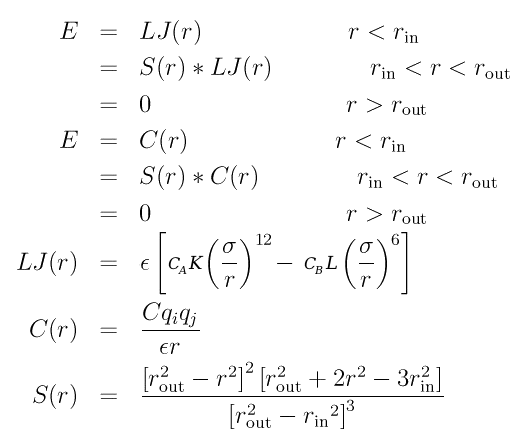Both the LJ and Coulombic terms require an inner and outer cutoff. They can be the same for both formulas or different depending on whether 2 or 4 arguments are used in the pair_style command. In each case, the inner cutoff distance must be less than the outer cutoff. It it typical to make the difference between the 2 cutoffs about 1.0 Angstrom.

### Coefficients

Compared to lj/charmm/coul/charmm , pair_style lj/charmm/coul/charmm/inter uses a slightly more general formula for the Lennard Jones interaction ("LJ(r)"). In addition to epsilon and sigma parameters (ϵ and σ), which scale the energy and distance, two optional dimensionless constants K and L can be used to independently tune the short-range and long-range terms. (These parameters typically lie in the range from -1.0 to 1.0.) The two remaining dimensionless constants, Ca and Cb are determined by the optional coeff_style argument. (See below.) By default, Ca and Cb are both 4, and K and L are 1 and -1.)

With this pair style you have the option to specify a coeff_style argument, which effects the formula for the Lennard Jones forces as follows:
```coeff_style      meaning:                        required user coeffs:
---------------------------------------------------------------------------
ab      LJ(r)=      A / r^12   -   B / r^6,      A,B     specified by user
es      LJ(r)= 4*e*[  (s/r)^12 -     (s/r)^6]    e,s   (K,L inferred from sign)
es1-2   LJ(r)=   e*[  (s/r)^12 -   2*(s/r)^6]    e,s   (K,L inferred from sign)
eskl    LJ(r)=   e*[K*(s/r)^12 +   L*(s/r)^6]    e,s,K,L specified by user
esx     LJ(r)= 4*e*[  (s/r)^12 -     (s/r)^6]    e,s,U0 (r > rc, U0=barrier at r=0)
= U0 - k*r^2                               (r < rc, k & rc determined by e,s,U0)
esxl    LJ(r)= 4*e*[  (s/r)^12 -   L*(s/r)^6]    e,s,U0,L (r > rc, U0=barrier r=0)
= U0 - k*r^2                               (r < rc, k & rc determined by e,s,U0)

-------- additional (less popular) coeff_styles  --------------------------

es4-4   equivalent to es
esx4-4  equivalent to esx
esx44l  equivalent to esxl
es1k2l  LJ(r)=   e*[K*(s/r)^12 + 2*L*(s/r)^6]    e,s,K,L specified by user
es4k4l  LJ(r)= 4*e*[K*(s/r)^12 +   L*(s/r)^6]    e,s,K,L specified by user
esx1-2  LJ(r)=   e*[  (s/r)^12 -   2*(s/r)^6]    e,s,U0 (K,L inferred from sign)
= U0 - k*r^2                        (r < rc, k & rc determined by e,s,U0)
esx12l  LJ(r)=   e*[  (s/r)^12 - 2*L*(s/r)^6]    e,s,U0,L specified by user
= U0 - k*r^2                        (r < rc, k & rc determined by e,s,U0,L)
esx11l  LJ(r)=   e*[  (s/r)^12 +   L*(s/r)^6]    e,s,U0,L specified by user
= U0 - k*r^2                        (r < rc, k & rc determined by e,s,U0,L)
---------------------------------------------------------------------------
```
Here "e" and "s" are shorthand for epsilon (ϵ) and sigma (σ). The "es", "es4-4", "es1-2", "esx", "esx4-4", and "esx1-2" options allow the user to omit K and L. However, even in this case, users have some limited control over K and L by altering the sign of ϵ (epsilon) and σ (sigma):
```    e>0, s>0   -->   K=1, L=-1    (default, typical usage)
e>0, s<0   -->   K=1, L=0     (pure 1/r^12 repulsion)
e<0, s<0   -->   K=1, L=1     (rarely used)
e<0, s>0   -->   K=0, L=1     (pure 1/r^6 repulsion)
```
The default coeff style is "es". (This way, the syntax of the pair_coeff commands remains unchanged from the syntax used in other lennard-jones pair_styles (like "lj/charmm/coul/charmm"), and the behavior is the same for positive "e" and "s".) Interactions between unlike atom types are determined by mixing . (See below.)

By default, the following coefficients must be defined for each pair of atoms types via the pair_coeff command as in the examples above, or in the data file or restart files read by the read_data or read_restart commands, or by mixing as described below:

• epsilon (energy units)
• sigma (distance units)

The following parameters are optional:

• epsilon_14 (energy units)
• sigma_14 (distance units)
• epsilonDIF (energy units)

The optional epsilon_14 and sigma_14 parameters customize the interactions between the first and fourth atoms participating in (charmm-style) dihedral interactions (IE. "1-4 interactions"). The optional epsilonDIF and sigmaDIF parameters allow the user to customize the interactions between atoms, depending on which molecules they belong to. (By default, atoms in different molecules use the epsilonDIF and sigmaDIF parameters, but this can be overridden.)

If these parameters are unspecified, they are set to "epsilon" and "sigma" by default. (However you must specify epsilon_14 and sigma_14 if you wish to specify the epsilonDIF and sigmaDIF parameters. They can not be omitted.)

No pair-specific cutoffs are specified because this CHARMM force field does not allow varying cutoffs for individual atom pairs; all pairs use the global cutoff(s) specified in the pair_style command.

#### Using alternate Lennard-Jones parameter conventions (coeff_style)

Again, there are several alternate conventions used to describe the Lennard-Jones interaction. These are listed in the table above. Some of these styles require additional parameters. For example, if you use the "eskl" "es4k4l" or "es1k2l" coeff styles, then you must specify these parameters:

• sigma (distance units)
• epsilon (energy units)
• K (dimensionless parameter)
• L (dimensionless parameter)
• sigma_14 (distance units)
• epsilon_14 (energy units)
• K_14 (dimensionless parameter)
• L_14 (dimensionless parameter)
• sigma_DIF (distance units)
• epsilon_DIF (energy units)
• K_DIF (dimensionless parameter)
• L_DIF (dimensionless parameter)
In this example:
```pair_coeff * *  0.40 3.6 1 -1  0 0 0 0  0.8 3.6 1 0
```
...the user has turned OFF the attractive part of the interaction between atoms in different molecules (by switching the "L" parameter to zero), while leaving the repulsive steric interaction in place. (The 1-4 interactions, "0 0 0 0", were set to zero in this case. As explained below, these parameters are ignored unless you are using dihedral_style charmm . But you must specify them.)

If you use the "esxl", "esx12l", "esx11l", or "esx44l" coeff styles, then you can replace the "K" parameter with "U0". "U0" is the energy barrier for particle overlap at small radii (U0). (To allow particle overlap, you must specify the "rsoftcore" parameter in the pair_style. See below.) In that case, the list of parameters is:

• sigma (distance units)
• epsilon (energy units)
• U0 (energy barier height)
• L (dimensionless parameter)
• sigma_14 (distance units)
• epsilon_14 (energy units)
• U0_14 (energy barier height)
• L_14 (dimensionless parameter)
• sigma_DIF (distance units)
• epsilon_DIF (energy units)
• U0_DIF (energy barier height)
• L_DIF (dimensionless parameter)
See the coeff_style table to see how these parameters are used. In this example:
```pair_coeff * *  0.4 3.6 1000.0 -1  0 0 0 0  0.4 3.6 2.0 -1
```
...the user has allowed atoms belonging to different molecules to pass through each other, by lowering the repulsive energy barrier to 2.0, while maintaining ordinary Lennard-Jones like behavior (by using a high-barrier of 1000) for particles in the same molecule. (The 1-4 interactions "0 0 0 0" were set to zero in this case.)
If you use the "esx" or "esx1-2" styles, then you can omit the "L" parameter:
• sigma (distance units)
• epsilon (energy units)
• U0 (energy barier height)
• sigma_14 (distance units)
• epsilon_14 (energy units)
• U0_14 (energy barier height)
• sigma_DIF (distance units)
• epsilon_DIF (energy units)
• U0_DIF (energy barier height)

Again, see the coeff_style table to see how these parameters are used. Again, all "1-4" interaction parameters must be explicitly set to 0.

### Optional "soft-core" interactions

(coeff_style esxl, esx12l, esx11l, esx44l, esx or esx1-2)
This pair style has an optional feature which allows users to weaken the repulsive forces at small radii.

The rsoftcore keyword tells LAMMPS to replace the Lennard-Jones interaction with U(r)=U0-k*r^2 at small radii, followed by a number which is an estimate (an upper bound) for the cutoff radius for the transition. This cutoff distance is not expressed in physical units like Angstroms (regardless of the "units" setting). Instead, this cutoff distance is expressed in multiples of sigma (which varies according to the atom types and mixing rules shown below). However this number is an upper bound only. (The actual cutoff distance is chosen in order to match the boundary conditions, and is written to the log file.) This parameter is typically set to 1.2. You can also use the pair_write command to verify that the transition occurs in a reasonable place.To use "soft-core" interactions, you must also use one of these coeff styles: esx, esx1-2, esx44l, esx12l, esx11l, esx4-4. The coeff_style you select specifies the syntax to use when specifying/interpreting the "U0" parameter. "U0" is the energy barrier for particle overlap, and it must be specified later in each "pair_coeff" command. (See discussion above.)

Note: LAMMPS will determine both the cutoff distance and "k" parameter automatically for each pair of atom types, in order to keep the energy and forces continuous at the boundary. (This information will be printed to the log file.)

Alternately, the rcore keyword allows you to use U(r)=U0+k/r^2 at small radii (instead of U(r)=U0-k*r^2). The "rcore" keyword must be followed by a cutoff distance which indicates where you want the transition to occur. It is typically set to 1.0 for coeff_style "es", "es4-4", or "es4k4l". In this case "1.0" sigma corresponds to the zero crossing radius, Repulsion at distances less than 1.0 sigma is typically very strong.) When coeff_style is set to "es1-2", or "es1k2l", then this ratio is typically set to 2^(-1/6), which is approximately 0.8909. (Again, this is expressed as a multiple of "sigma".)

The 1/r^2 approximation to the Lennard-Jones repulsive interaction at short distances is more numerically stable than a 1/r^12 interaction, and this can be a useful alternative to rRESPA (which is not supported by this pair-style).

Unfortunately, if you use either "rcore" or "rsoftcore", you must explicitly set all 1-4 coeffs to zero. (Otherwise, if you use "dihedral_style charmm", 1-4 interactions are not treated correctly at these short distances.) However this is rarely a restriction for most users, since 1-4 interactions are only calculated using these parameters if you also happen to be using dihedral_style charmm. Keep in mind that, 1-4 interactions are still calculated, (even if you set their coeffs to 0), and their weights can still be specified with the "special_bonds" command, just as they would be for any other pair_style. In that case the "epsilon", "sigma", "Kappa", "U0" and "Lambda" parameters are used (instead of "epsilon_14", "sigma_14", "K_14", "U0_14", and "L_14", which are ignored.) So setting these parameters to 0 has no effect. But you are still required to do set them to zero. (The 1-4 parameters discussed here are only present in order to remain backwards-compatible with pair_style lj/charmm/coul/charmm . These parameters are discussed in the documentation for that pair_style.)

Neither kinds of soft repulsion (1/r^2 or -r^2) are recommended when charges are present. Even 1/r^2 repulsion is typically not strong enough to prevent opposite charges from getting unrealistically close together.

Mixing, shift, table, tail correction, restart, rRESPA info:

For atom type pairs I,J and I != J, the epsilon, sigma, epsilon_14, and sigma_14, epsilonDIF, and sigmaDIF coefficients for all of the lj/charmm pair styles can be mixed. The default rule for mixing epsilon and sigma parameters is arithmetic to coincide with the usual settings for the CHARMM force field. See the "pair_modify" command for details.

The rule for mixing interactions between one or two repulsive particles is determined by the optional rmix_style argument. By default rmix_style is set to "maxmax". With this choice, repulsive interactions override attractive ones. The other choices are shown below:

```rmix_style      meaning:
-----------------------------------------------------------
maxmax --> Kij=MAX(Ki,Kj),  Lij=MAX(Li,Lj)  repulsive interactions "win"
maxmin --> Kij=MAX(Ki,Kj),  Lij=MIN(Li,Lj)
minmax --> Kij=MIN(Ki,Kj),  Lij=MAX(Li,Lj)
minmin --> Kij=MIN(Ki,Kj),  Lij=MIN(Li,Lj)
arith  --> Kij=0.5*(Ki+Kj), Lij=0.5*(Li+Lj)  (if coeff_style not "ab")
--> Aij=0.5*(Ai+Aj),  Bij=0.5*(Bi+Bj)  (if coeff_style is "ab")
mix_es_from_ab  -->  only available when coeff_style is "ab"
```
When using "softcore" interactions (U(r)=U0-k*r^2 for small r), the "U0" parameter replaces "K", but the mixing rules are the same:
```rmix_style      meaning:
-----------------------------------------------------------
maxmax --> U0ij=MAX(U0i,U0j),  Lij=MAX(Li,Lj)  repulsive interactions "win"
maxmin --> U0ij=MAX(U0i,U0j),  Lij=MIN(Li,Lj)
minmax --> U0ij=MIN(U0i,U0j),  Lij=MAX(Li,Lj)
minmin --> U0ij=MIN(U0i,U0j),  Lij=MIN(Li,Lj)
arith  --> U0ij=0.5*(U0i+U0j), Lij=0.5*(Li+Lj)  (if coeff_style not "ab")
```
None of these rmix_style settings effect the mixing rules for epsilon and sigma ("e" and "s"). (They are still determined by the preferences set by the "pair_modify" command.)
(Comment: Feel free to suggest other mixing/combination rules I should add to this list. I admit, these rules are ad-hoc.)

None of the lj/charmm pair styles support the pair_modify shift option, since the Lennard-Jones portion of the pair interaction is smoothed to 0.0 at the cutoff.

None of the lj/charmm pair styles support the pair_modify tail option for adding long-range tail corrections to energy and pressure, since the Lennard-Jones portion of the pair interaction is smoothed to 0.0 at the cutoff.

All of the lj/charmm pair styles write their information to binary restart files, so pair_style and pair_coeff commands do not need to be specified in an input script that reads a restart file.

This style only supports the pair keyword of run_style respa. See the run_style command for details.

 Shaded regions indicate which pairs of molecules use "DIF" parameters. (This example has 8 molecules.) LAMMPS command```pair_style lj/charmm/coul/charmm/inter 8.5 9.0 both 2*4 6*7 # Interactions between atoms from molecules 2,3,or4 # with atoms from molecules 6 or 7 use "DIF" params ``````pair_style lj/charmm/coul/charmm/inter 8.5 9.0 both 3*5 3*5 # Interactions between atoms from molecules 3,4,or5 # with atoms from molecules 3,4,or5 use "DIF" params ``````pair_style lj/charmm/coul/charmm/inter 8.5 9.0 both 3*5 3*5 mi!=mj # Same rules as the example above. # However atoms in the same molecule # do NOT use DIF parameters ```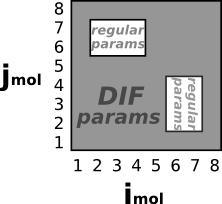```pair_style lj/charmm/coul/charmm/inter 8.5 9.0 nboth 2*4 6*7 # Interactions between atoms in molecules 2,3,or 4 # and molecules 6 or 7 use ordinary params. # All other interactions use "DIF" parameters. ```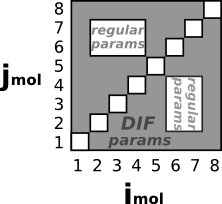```pair_style lj/charmm/coul/charmm/inter 8.5 9.0 nboth 2*4 6*7 mi!=mj # Same rules as the example above. # However atoms in the same molecule # do not use DIF parameters ```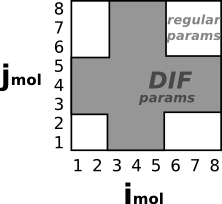```pair_style lj/charmm/coul/charmm/inter 8.5 9.0 either 3*5 #Use "DIF" params if either atom has 3<=molID<=5 ``````pair_style lj/charmm/coul/charmm/inter 8.5 9.0 either 3*5 mi!=mj # Same rules as the example above. # However atoms in the same molecule # do NOT use DIF parameters ```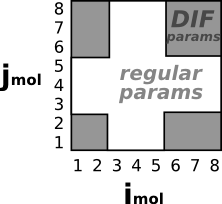```pair_style lj/charmm/coul/charmm/inter 8.5 9.0 neither 3*5 #Use "DIF" params if neither atom has 3<=molID<=5 ```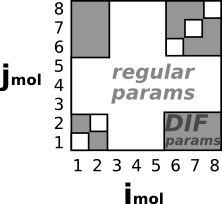```pair_style lj/charmm/coul/charmm/inter 8.5 9.0 neither 3*5 mi!=mj # Same rules as the example above. # However atoms in the same molecule # do NOT use DIF parameters ``````pair_style lj/charmm/coul/charmm/inter 8.5 9.0 eskl nucleate 1 # Atoms in different molecules use (typically repulsive) "DIF" parameters # UNLESS one of them belongs to molecule 1. Note: This is equivalent to: # pair_style lj/charmm/coul/charmm/inter 8.5 9.0 eskl neither 1 mi!=mj pair_coeff 1 1 0.4 3.6 4 -4 0.4 3.6 4 -4 0.4 3.6 4 0 # Atoms of type 1 in DIFFERENT molecules use "DIF" params: "0.4 3.6 4 0", which # are purely repulsive ("0.4 3.6 4 -4" are used for ordinary and 1-4 interactions) fix 1 all renum/mol 1000 type 4* 4* rmax 5.0 nucleate 1 # Every 1000 iterations reassign atoms to molecules (molecule-ID numbers) according # to how close they are together. Atoms (of type >= 4) which lie within a distance # of 5.0 are assigned to the same molecule-ID. This keeps track of which atoms are # in each cluster. These settings prevent cluster-cluster aggregation. ```